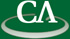## Eventual Positivity of Rational Function Coefficients.

### Tuesday March 6th at 1:30pm in K9509.

```Abstract

Given a univariate rational function F(x), a classic problem in
combinatorics and theoretical computer science is to determine whether the
power series coefficients of F are eventually strictly positive.  The
decidability of positiveness, and of determining when the power series of F
has a 0 coefficient, is still open after many decades of study (a state of
affair described by Terry Tao as "faintly outrageous" and a "mathematical
embarrassment" by Dick Lipton).  Likewise, given a multivariate rational
function it is of interest to determine when only a finite number of its
power series coefficients are non positive.  In this talk we examine the
problem through the use of asymptotic expansions of rational function
coefficients, settling some conjectures of Straub and Zeitlin.
```